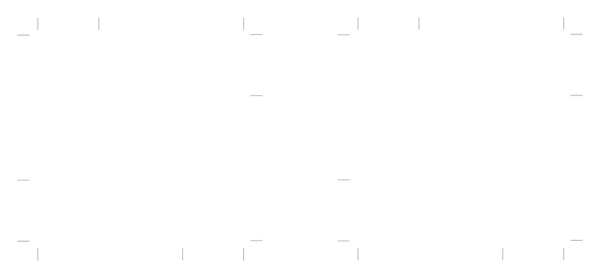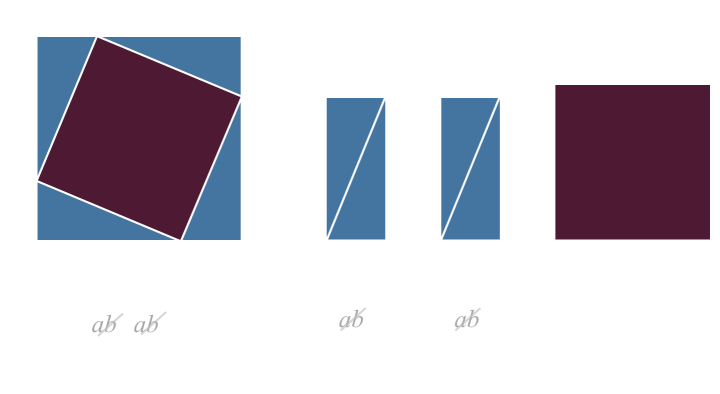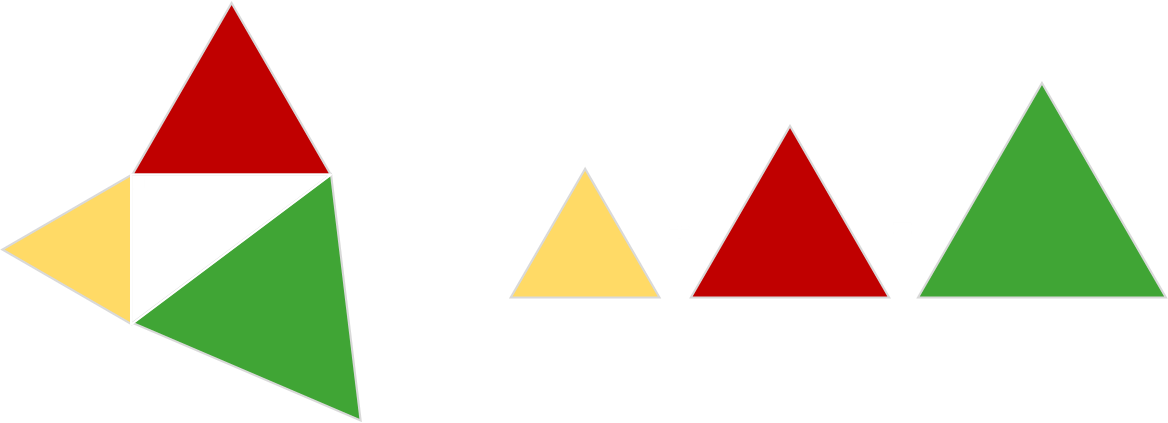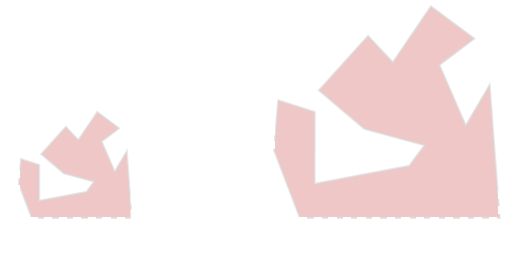# Pythagoras Revisited

In Euclidean geometry, one of the most well-known theorems is the Pythagorean theorem. It states that, in a right-angle triangle, the square of the hypotenuse is equal to the sum of the squares of the other two sides.
For a right triangle with hypotenuse c, and other sides a, b the equation states:There are dozens of ways to prove the theorem, but a simple one is a geometric rearrangement.Starting with a line of length a+b, we can make four copies of this to make a square.Connecting the points a away from the corners makes another square, internally, with side c. This also forms four right-angle triangles.
This composition can be rearranged to make two rectangles of area ab and the square c2. The two configurations are the same total area. A little bit of algebra confirms the theorem.## Areas

You've probably seen the formula represented graphically by placing squares on each edge of the triangle. As the area of each square is the edge length squared, there is a simple relationship between the areas of the squares.There's even some fabulous physical demonstrations of this, as seen in this video:

## Other Shapes

What might blow your mind is that it also works for Pentagons.Or triangles …Or any similar shape …## Why?

The answer is pretty simple. When you scale up any shape a linear dimension, its area scales up by the square of that change.It seems obvious now, when you think about it, but the first time seen it is a surprise to many.
Stay safe.